# Thread: Integrating fractions

1.Originally Posted by Krizalidthe general trick is to complete the square and then perform a trig. substitution.
For example, integrating

$\displaystyle \displaystyle{\frac{1}{x^2 + x + 1}}$

we would transform it so...

$\displaystyle \ =\ \displaystyle{\frac{1}{(x + \frac{1}{2})^2 + \frac{3}{4}}}$

(square now completed). Then...

$\displaystyle \ =\ \displaystyle{\frac{1}{\frac{3}{4}[\frac{4}{3}(x + \frac{1}{2})^2 + 1]}}$

$\displaystyle \ =\ \displaystyle{\frac{4}{3}\ \frac{1}{(\frac{2}{\sqrt{3}}x + \frac{1}{\sqrt{3}})^2 + 1}}$

It's worth noticing that whether or not there are any incomplete squares to complete, a crucial step often is what came after in this case, which is turning the constant term into a one so you're able to do a trig sub and then use a Pythag identity. E.g., no completing the square here...

$\displaystyle \displaystyle{\frac{1}{3 + x^2}}$

... but you want to turn the 3 into a one in the same way as with the three quarters, above.

Spoiler:
$\displaystyle \ =\ \displaystyle{\frac{1}{3(1 + \frac{1}{3}x^2)}}$

$\displaystyle \ =\ \displaystyle{\frac{1}{3}\ \frac{1}{1 + (\frac{1}{\sqrt{3}}x)^2}}$

Then the trig sub, which is really a matter of identifying $\displaystyle \ \frac{1}{\sqrt{3}}x\$ as an inner function of a composite, and reasoning that if we swap the whole of this inner function for tan, we'll be able to integrate with respect to the inner-most variable. Just in case a picture helps...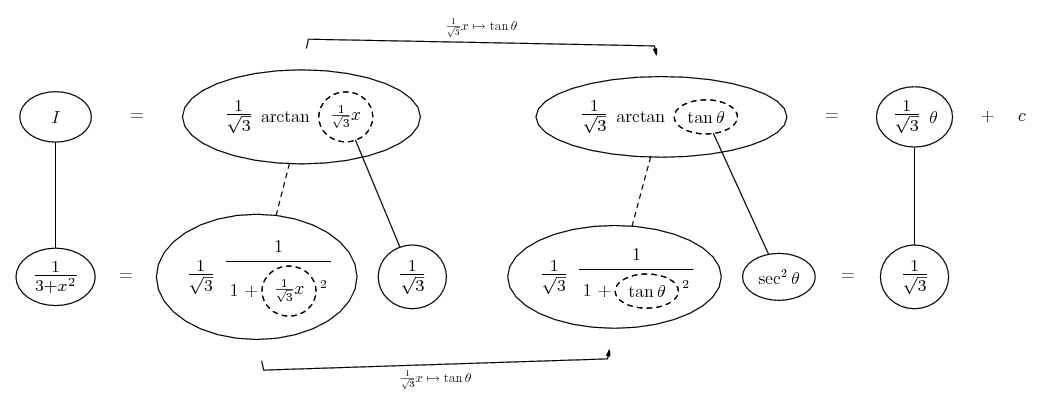... where (key in spoiler) ...

Spoiler:... is the chain rule. Straight continuous lines differentiate downwards (integrate up) with respect to the main variable (in this case theta), and the straight dashed line similarly but with respect to the dashed balloon expression (the inner function of the composite which is subject to the chain rule).

The general drift is...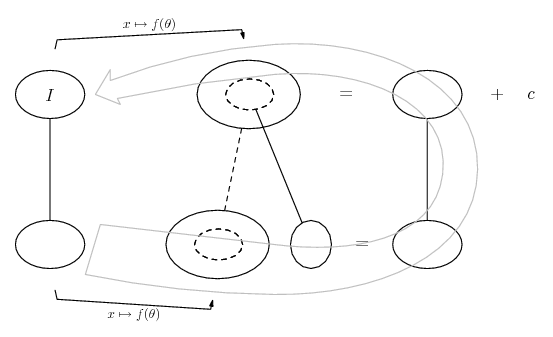For the 'completed' square...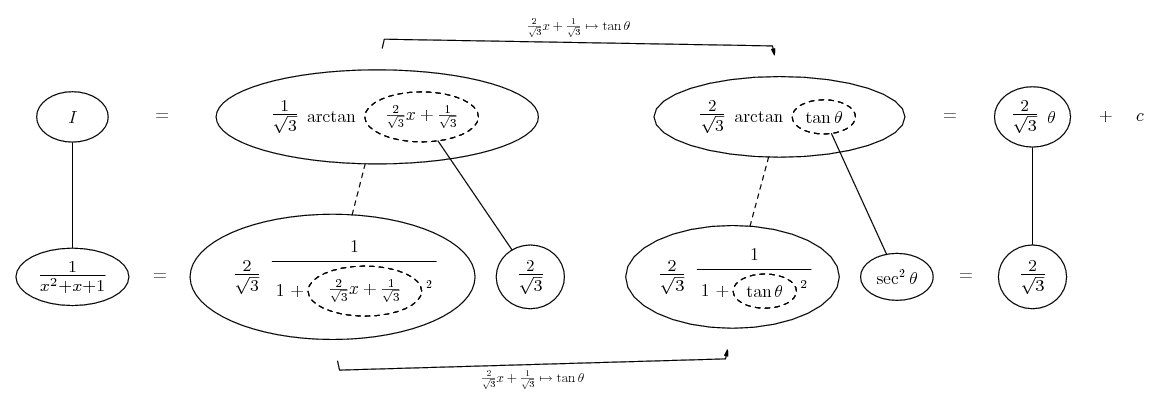If you've memorised $\displaystyle \ \frac{1}{1 + x^2}\$ as a standard integrand, of course, you won't need to map to or substitute with tan...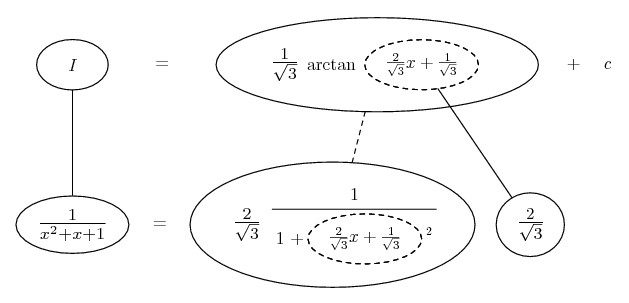PS...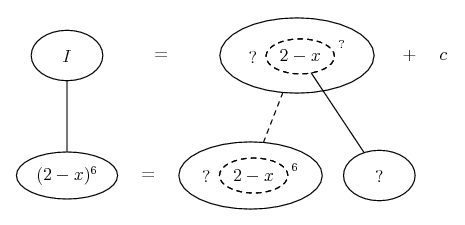Spoiler: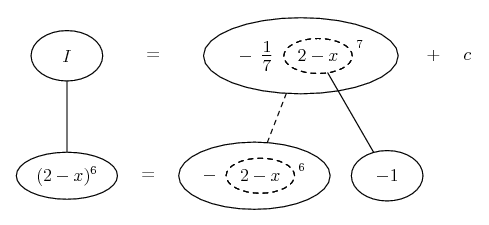_________________________________________

Don't integrate - balloontegrate!

Balloon Calculus; standard integrals, derivatives and methods

Balloon Calculus Drawing with LaTeX and Asymptote!

2. Thanks to everyone! This helps immensely, I really appreciate it. So for a function like (1 - x^2)^1/2 from 0 to 1, what I would do is make u = 1 - x^2, right? And then I would make it du/dx, giving me -2x..from here I'm less sure what to do. Do I try and solve for dx, or x, or what?

3.Originally Posted by bobsanchezThanks to everyone! This helps immensely, I really appreciate it. So for a function like (1 - x^2)^1/2 from 0 to 1, what I would do is make u = 1 - x^2, right? And then I would make it du/dx, giving me -2x..from here I'm less sure what to do. Do I try and solve for dx, or x, or what?
to solve integration of irrational functions ...

$\displaystyle \displaystyle I_1=\int R(x,\sqrt[n]{ax+b} ) \; dx$
$\displaystyle \displaystyle I_2=\int R(x,\sqrt[n]{\frac{ax+b}{cx+d}})\; dx$

first step :

substitution $\displaystyle \displaystyle ax+b=t^n$ or for second $\displaystyle \displaystyle \frac{ax+b}{cx+d}= t^n$

second step :

calculating "dx" :

$\displaystyle \displaystyle dx=\frac {nt^{n-1}}{a}\; dt$ or for second $\displaystyle \displaystyle dx = \frac{(ad-bc)^2 nt^{n-1}}{(d-b)(a-ct^n)}\; dt$

third step :

express "x"

$\displaystyle \displaystyle x=\frac{t^{n}-b}{a}$

or for second

$\displaystyle \displaystyle x=\frac{dt^{n}-b}{a-ct^n}$

4th step :

Calculating integralP.S. here's one simple example$\displaystyle \displaystyle \int \frac {dx}{(x+1)\sqrt{1-x}}$

$\displaystyle 1-x=t^2 \Rightarrow x=1-t^2$

$\displaystyle dx=-2tdt$

$\displaystyle \displaystyle \int \frac {dx}{(x+1)\sqrt{1-x}}= \int \frac {-2tdt}{(1-t^2+1)t}=$

$\displaystyle \displaystyle = -2 \int \frac {dt}{2-t^2} = 2\int \frac {dt}{t^2-2} =$

$\displaystyle \displaystyle =2 \int \frac {dt}{(t-\sqrt{2})(t+\sqrt{2})}= 2 \frac {1}{2\sqrt{2}} \ln {\Big{|}\frac {t-\sqrt{2}}{t+\sqrt{2}}\Big{|}}=$

$\displaystyle \displaystyle = \frac {1}{\sqrt{2}} \ln {\Big{|}\frac {\sqrt{1-x}-\sqrt{2}}{\sqrt{1-x}+\sqrt{2}}\Big{|}} + C$4. You guys are awesome. Again, to check myself: the integral of (4 + 3x)^1/2, evaluated from 0 to 7.

I make u = 4 + 3x, and find that dx = 1/3

I get 1/3 times [2(4 + 3x)^3/2]/3

After evaluating, one gets 50/9, or 5.56. Is this correct?

5. $\displaystyle \int{(4 + 3x)^{\frac{1}{2}}\,dx} = \frac{1}{3}\int{3(4+3x)^{\frac{1}{2}}\,dx}$

Let $\displaystyle u = 4 + 3x$ so that $\displaystyle du = 3\,dx$ and the integral becomes

$\displaystyle \frac{1}{3}\int{u^{\frac{1}{2}}\,du}$

$\displaystyle = \frac{1}{3}\left(\frac{u^{\frac{3}{2}}}{\frac{3}{2 }}\right) + C$

$\displaystyle = \frac{1}{3}\left(\frac{2u^{\frac{3}{2}}}{3}\right) + C$

$\displaystyle = \frac{2u^{\frac{3}{2}}}{9} + C$

$\displaystyle = \frac{2(4 + 3x)^{\frac{3}{2}}}{9} + C$.

That means

$\displaystyle \int_0^7{(4+3x)^{\frac{1}{2}}\,dx} = \left[\frac{2(4 + 3x)^{\frac{3}{2}}}{9}\right]_0^7$

$\displaystyle = \left[\frac{2(4 + 3\cdot 7)^{\frac{3}{2}}}{9}\right] - \left[\frac{2(4+3\cdot 0)^{\frac{3}{2}}}{9}\right]$

$\displaystyle = \frac{2\cdot 25^{\frac{3}{2}}}{9} - \frac{2\cdot 4^{\frac{3}{2}}}{9}$

$\displaystyle = \frac{250}{9} - \frac{16}{9}$

$\displaystyle = \frac{234}{9}$

$\displaystyle = 26$.

#### Search Tags

fractions, integrating